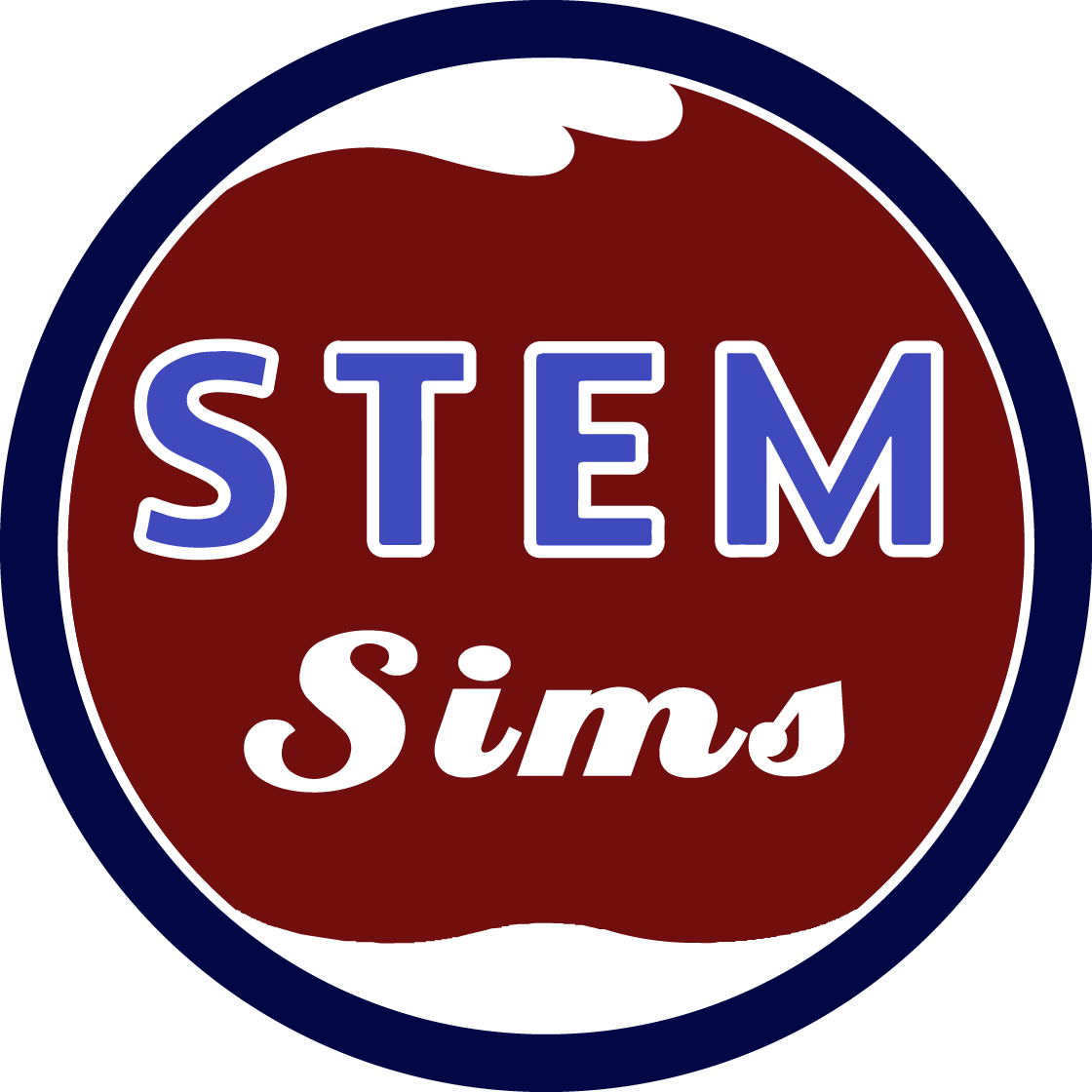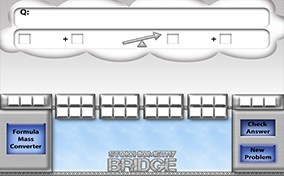# Stoichiometry BridgeEvery chef requires a recipe for a favorite dish that states how much of each ingredient is required to make a certain amount of that food. Scientists also used a recipe, called a balanced chemical equation, to make a certain amount of product. Can you create and use a chemical recipe to make a desired product? Get “cooking” doing some stoichiometry.

### What is stoichiometry?

Stoichiometry is a mathematical method used to calculate the amount of reactants and products required in a chemical reaction. The amount of reactants and products calculated can be measured in units of mass, volume, number of moles, or the number of particles.

### What is a conversion factor?

A conversion factor is a mathematical term that allows the one unit of measurement to be changed into another unit of measurement. A conversion factor must be a true and correct statement that relates the two units of measurement. The factor is a fraction where the numerator is one unit of measurement and the denominator is a different unit of measurement with equal value.

### What is the factor label method?

Since some calculations require multiple steps, methods are helpful to complete the process. The factor label method is one such tool that uses conversion factors in the form of fractions to change from one unit of measurement to another. Each conversion factor is lined up in a sequence so that the units of the first term in the numerator are cancelled out with the conversion factor fraction in the denominator of the next term. This process repeats until the final desired unit only remains in the numerator.

### What are some tips for doing stoichiometry?

One of the most important tips is to always start all chemical calculations with the balanced chemical equation. This implies that you first must correctly write the chemical formula of all reactants and products in the reaction. Any errors in writing the chemical formulas or balancing the equation will result in the propagation of errors throughout your calculation. A second tip is to create a plan that states what conversion factors are required to complete your calculation. Next, always start your calculation with what is given in the problem, and then place the unit of the given quantity in the denominator of the next calculation factor. The numerator of the next factor should contain the unit of where you want to go with your next calculation. Continue with this process, cancelling out units until the only remaining unit is the one that you are trying to find with your calculation.

### What is a mole?

A mole is a specific quantity of things. A mole is the number of things that has the same number of particles found in 12 grams of the carbon-12 isotope. Since atoms are very tiny and lots of them fit in a very small space, a large unit of measure was created that allowed people to talk about many atoms…the mole. One mole of things equals 6.02 X 1023 of those things, which is a whole lot of things! So, one mole of gold atoms would contain 6.02 X 1023 atoms of gold.

### What is gram atomic mass?

The gram atomic mass of an atom is the mass of one mole of the atom measured in grams. This value is found on the Periodic Table (usually listed on the table as the atom’s atomic mass).

### What is gram formula mass?

The gram formula mass is the mass of one formula unit measured in grams. To find this value, the number of moles of each type of atom in a formula is multiplied by its gram atomic mass. Each of these products is then summed to yield the gram formula mass.

### What are some common stoichiometry mistakes?

The top ten mistakes students make when using stoichiometry include:
1. Writing the chemical formula of the reactants and products incorrectly,
2. Not balancing the chemical equation,
3. Writing incorrect conversion factors, like saying 1 inch equals 12 feet instead of 1 foot equals 12 inches,
4. Not including a unit of measurement with each numerical quantity,
5. Not making sure all of the units of measurement except the desired unit cancel out,
6. Forgetting that measured quantities require significant figure considerations,
7. Not knowing the difference between gram formula mass, formula mass, atomic mass, and the gram atomic mass,
8. Not remembering the 7 diatomic elements (H, O, F, Br, I, N, and Cl),
9. Not including the mole ratio when needed, and
10. Not using Avogadro’s number when converting from moles to particles.

## Lessons

You need to log in to access this simulation.